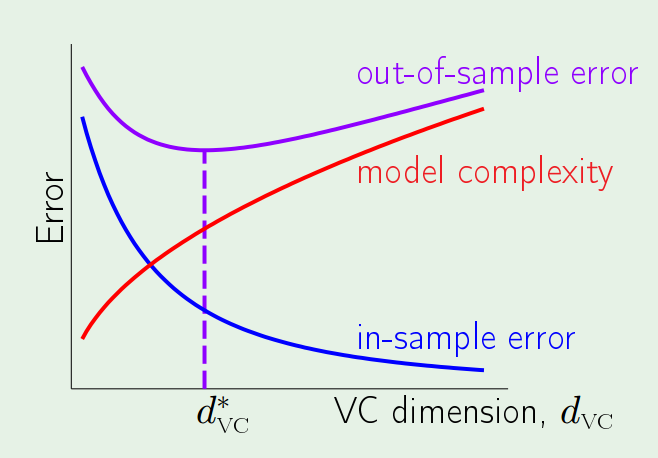# Learning from Data 4: Error and Noise

Learning from Data is a Machine Learning MOOC taught by Caltech Professor Yaser Abu-Mostafa. I took the course in 2014 through edX. It was a great course, well-organized and into the details.

Recently I have been reviewing the notes and the summary of the course, and I thought I might just post them here. All my code used for the homework can be found at https://github.com/Chaoping/Learning-From-Data.

Lecture 4: Error and Noise

What is error? We wish to learn a hypothesis that approximates the target function, “$h \approx f$”. The error basically reflects how a hypothesis does the job. And we call it: “$E(h,f)$”. Then the pointwise error for any point can be defined as: $$\mathrm{e}(h(\mathbf{x}), f(\mathbf{x}))$$

If we consider the overall $E(h,f)$ error as the average of the pointwise error, we have:

in-sample error: $$E_{in}(h) = \frac{1}{N}\sum_{n=1}^{N}{\mathrm{e}(h(\mathbf{x}_n), f(\mathbf{x}_n))}$$

out-of-sample error: $$E_{out}(h) = \mathbb{E}_\mathbf{x}[\mathrm{e}(h(\mathbf{x}), f(\mathbf{x}))]$$

But how do we measure the error? The error measure should be specified by the user. However such thing isn’t always possible. So often we take alternatives that are:

• Plausible, meaning the measure makes sense or at least intuitively correct. For example the squared error is equivalent to Gaussian noise.
• Friendly, meaning it is convex so that the learning algorithm can work effectively, or it may even have a closed-form solution just like the squared error.

With the error measure defined, we then can have a learning algorithm to pick $g$ out of $H$.

Now, let’s reconsider about target function – is it really a function? No, because very possible that two identical input may have different output. So instead, we have a “target distribution”: $$P(y|\mathbf{x})$$ Each point is generated from the joint distribution of $$P(\mathbf{x})P(y|\mathbf{x})$$

And the “noisy target” can be seen as a combination of a deterministic target: $$f(\mathbf{x}) = \mathbb{E}(y|\mathbf{x})$$ plus the noise: $$y-f(\mathbf{x})$$

Finally, the learning diagram shall look like this:At this point, we know:

Learning is feasible. It is likely that $$E_{in}(g) \approx E_{out}(g)$$

What we are trying to achieve is that $g \approx f$, or equivalently $$E_{out}(g) \approx 0$$

So learning is split into 2 goals that have to be achieved at the same time:

• $E_{in}(g)$ must be close to $E_{out}(g)$.
• $E_{in}(g)$ must be small enough.

This leads to the following topics, where the model complexity will play a big role affecting both. The trade-off lies that the more complex a model is, the smaller $E_{in}(g)$ will be and at the same time it loses its ability to track $E_{out}(g)$.## Author: Chaoping Guo

Learning is a privilege that I am grateful for.